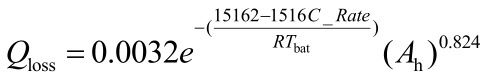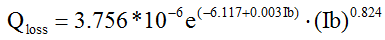# How to solve or avoid the problem of “{log-affine} .* {concave}”?

Hi,I would like to achieve the following expression in CVX,where C_Rate and Ah are vectors(1400×1),R and Tbat are constants.We know exp() is convex,but x.^0.824 is concave.Therefore,It will prompt for errors in Matlab:
%Cannot perform the operation:
% {log-affine} .
{concave}
So is there any ways to solve or avoid this error in Matlab?Thanks to everyone for their help.I mean how to implement this expression in CVX fitting DCP rule?Can you give me a reply?Thank you~:sweat: